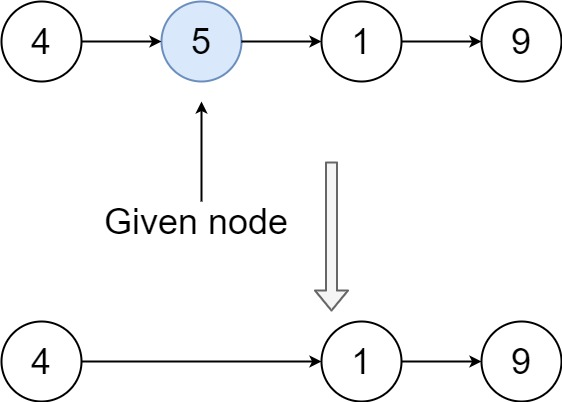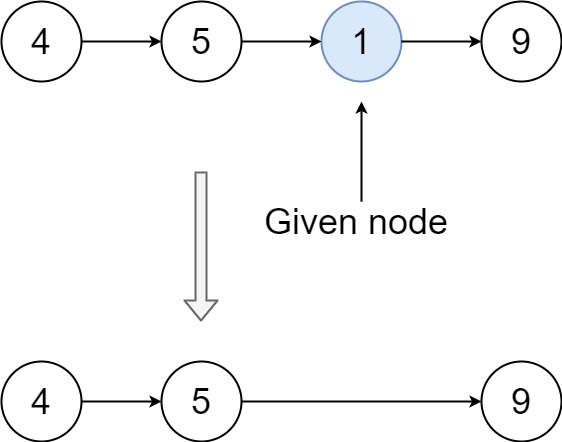## Algorithm

Problem Name: 237. Delete Node in a Linked List

There is a singly-linked list `head` and we want to delete a node `node` in it.

You are given the node to be deleted `node`. You will not be given access to the first node of `head`.

All the values of the linked list are unique, and it is guaranteed that the given node `node` is not the last node in the linked list.

Delete the given node. Note that by deleting the node, we do not mean removing it from memory. We mean:

• The value of the given node should not exist in the linked list.
• The number of nodes in the linked list should decrease by one.
• All the values before `node` should be in the same order.
• All the values after `node` should be in the same order.

Custom testing:

• For the input, you should provide the entire linked list `head` and the node to be given `node`. `node` should not be the last node of the list and should be an actual node in the list.
• We will build the linked list and pass the node to your function.
• The output will be the entire list after calling your function.

Example 1:```Input: head = [4,5,1,9], node = 5
Output: [4,1,9]
Explanation: You are given the second node with value 5, the linked list should become 4 -> 1 -> 9 after calling your function.
```

Example 2:```Input: head = [4,5,1,9], node = 1
Output: [4,5,9]
Explanation: You are given the third node with value 1, the linked list should become 4 -> 5 -> 9 after calling your function.
```

Constraints:

• The number of the nodes in the given list is in the range `[2, 1000]`.
• `-1000 <= Node.val <= 1000`
• The value of each node in the list is unique.
• The `node` to be deleted is in the list and is not a tail node.

## Code Examples

### #1 Code Example with C Programming

```Code - C Programming```

``````
void deleteNode(struct ListNode* node) {
struct ListNode *p;

if (!node || !node->next) return;

p = node->next;
*node = *p;
free(p);
}
``````
Copy The Code &

Input

cmd
head = [4,5,1,9], node = 5

Output

cmd
[4,1,9]

### #2 Code Example with Javascript Programming

```Code - Javascript Programming```

``````
const deleteNode = function(node) {
if (node.next !== null) {
node.val = node.next.val;
node.next = node.next.next;
}
};
``````
Copy The Code &

Input

cmd
head = [4,5,1,9], node = 5

Output

cmd
[4,1,9]

### #3 Code Example with Python Programming

```Code - Python Programming```

``````
class Solution:
def deleteNode(self, node):
node.val = node.next.val
node.next = node.next.next
``````
Copy The Code &

Input

cmd
head = [4,5,1,9], node = 1

Output

cmd
[4,5,9]

### #4 Code Example with C# Programming

```Code - C# Programming```

``````
namespace LeetCode
{
{
public void DeleteNode(ListNode node)
{
node.val = node.next.val;
node.next = node.next.next;
}
}
}
``````
Copy The Code &

Input

cmd
head = [4,5,1,9], node = 1

Output

cmd
[4,5,9]